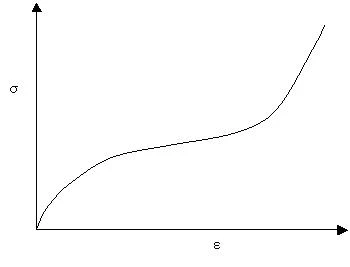Glossary

# Elasticity and Modulus of Elasticity

Rubber elasticity or entropy elasticity describes the resistance of any rubber or elastomer system against an externally applied deformation or StrainStrain describes a deformation of a material, which is loaded mechanically by an external force or stress. Rubber compounds show creep properties, if a static load is applied.strain

Rubber elasticity is related to reversible changes in entropy. The origin of these reversible changes of entropy within a rubber or elastomer matrix along the polymer chains can be described by the following aspects:

If a zig-zag elastomer molecule is extended due to any external force, the bonding angle between neighborhood molecules along the chain will be changed. If these small changes take place without the activation of friction processes, no deformation energy is required.
On the other hand, stretching means orientation takes place on a molecular basis and entropy (disorder within a system) will decrease.
If the external force is removed, thermal energy from the environment will be absorbed and the molecules return to their initial orientation; the bonding angle will return and the molecules will shrink to their original shape.

An Elastic modulusThe complex modulus (elastic component), storage modulus, or G’, is the “real” part of the samples the overall complex modulus. This elastic component indicates the solid like, or in phase, response of the sample being measurement. elastic modulus (also known as modulus of elasticity) is a quantity that measures an object or substance's resistance to being deformed elastically (i.e., non-permanently) when a StressStress is defined as a level of force applied on a sample with a well-defined cross section. (Stress = force/area). Samples having a circular or rectangular cross section can be compressed or stretched. Elastic materials like rubber can be stretched up to 5 to 10 times their original length.stress is applied to it. The elastic modulus of an object is defined as the slope of its StressStress is defined as a level of force applied on a sample with a well-defined cross section. (Stress = force/area). Samples having a circular or rectangular cross section can be compressed or stretched. Elastic materials like rubber can be stretched up to 5 to 10 times their original length.stress-strain curve in the elastic deformation region: A stiffer material will have a higher elastic modulus. The elastic modulus is defined according to the following formula:Figure 1: The figure shows a typical stress-strain curve for rubber elasticity.

The E modulus can be measured with the DMA 242 Artemis® and DMA EPLEXOR®®.